# (1 point) xi(t) Let x(t) = be a solution to the system of differential equations: x2(t)...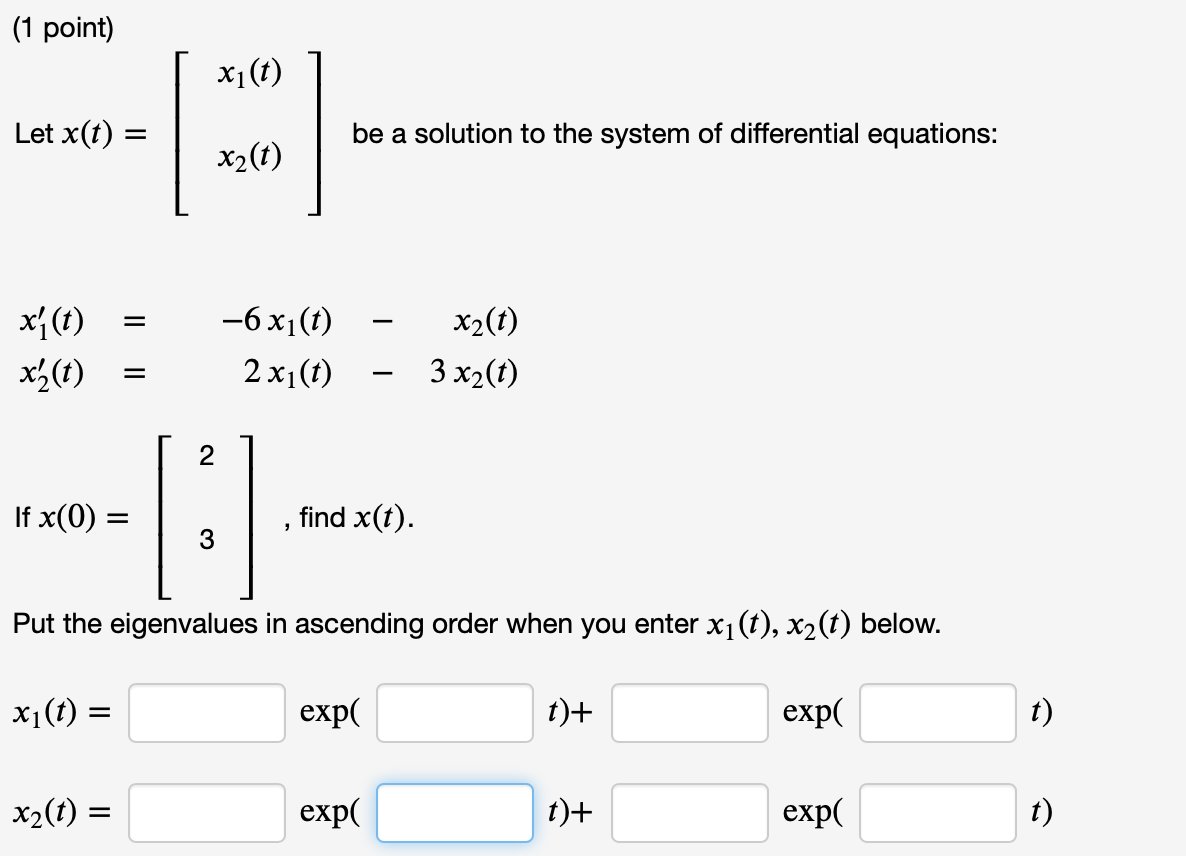(1 point) xi(t) Let x(t) = be a solution to the system of differential equations: x2(t) xy(t) x'z(t) –6 x (1) 2 xi(t) x2(t) 3 x2(t) = If x(0) find x(t). 2 3 Put the eigenvalues in ascending order when you enter xi(t), x2(t) below. xi(t) = exp( t)+ expo t) x2(t) = exp( t)+ expl t)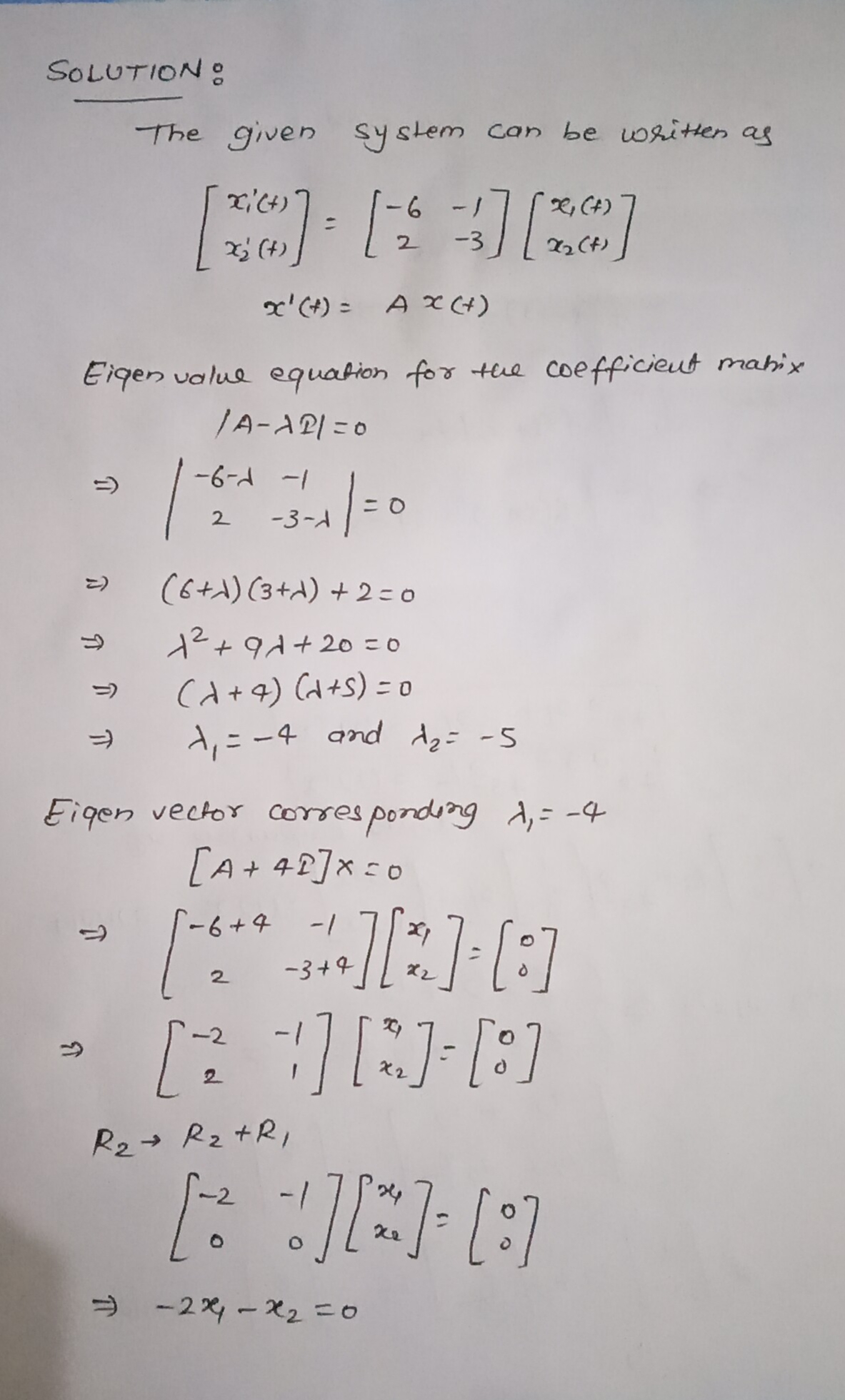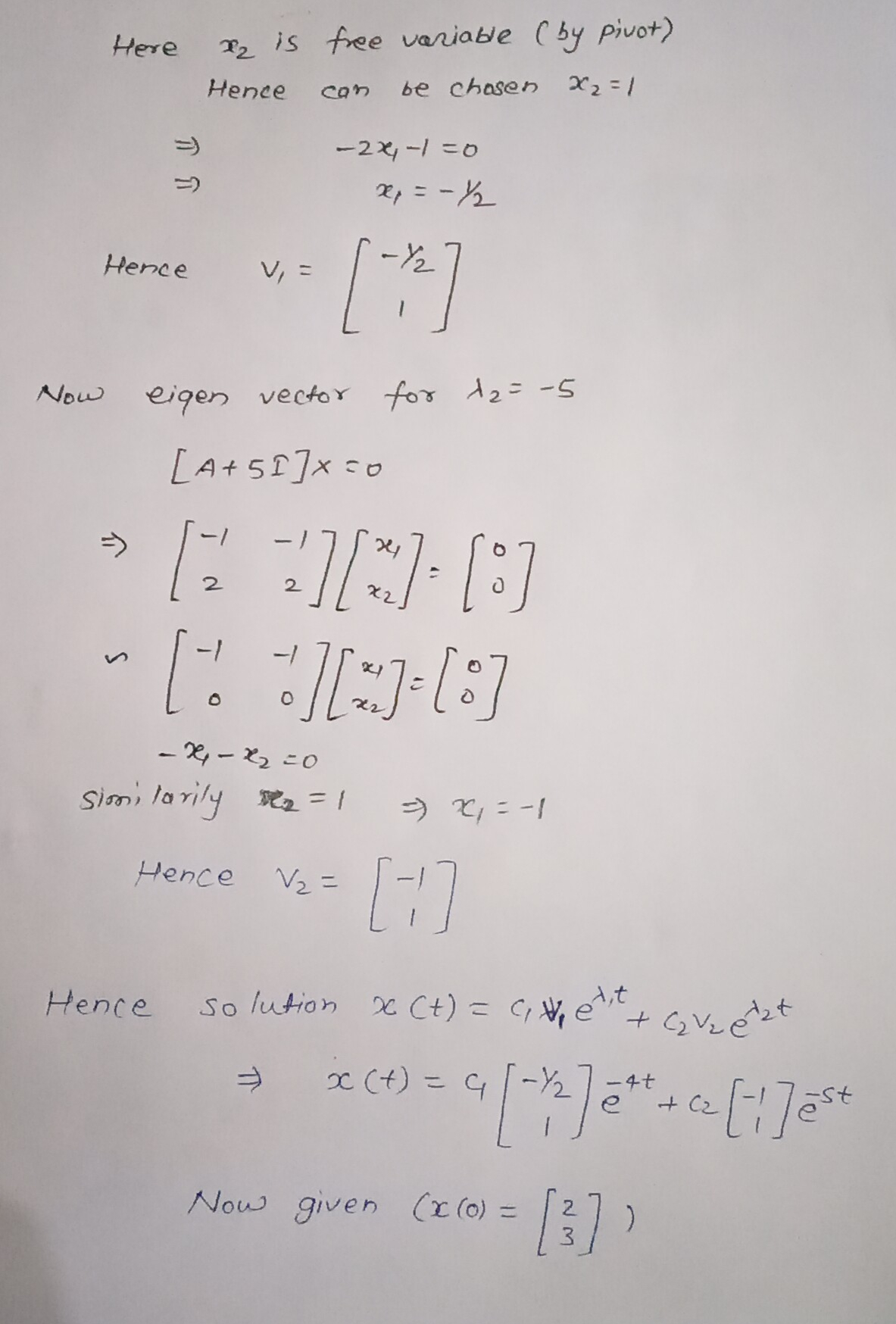##### Add Answer of: (1 point) xi(t) Let x(t) = be a solution to the system of differential equations: x2(t)...
Similar Homework Help Questions
• ### (1 point) 21(t) Let X(t) = be a solution to the system of differential equations: 22(t)...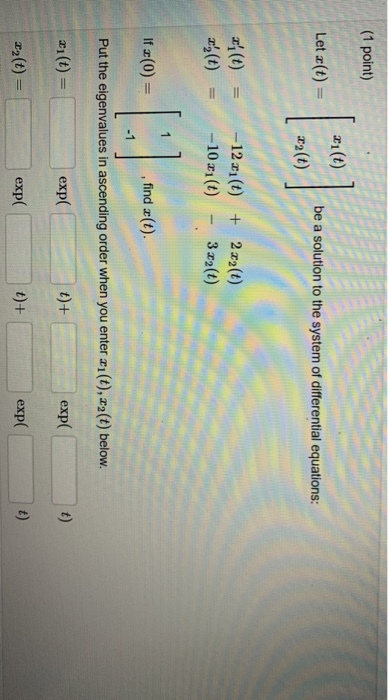(1 point) 21(t) Let X(t) = be a solution to the system of differential equations: 22(t) (t) x',(t) - 12x1() + 2 x2(t) -10 x1(0) 3 x2(t) If x(0) [:] find (t). Put the eigenvalues in ascending order when you enter xi(t), 22(t) below. * (t) = exp( t)+ expo t) 22(t) exp( t)+ exp( t)

• ### (1 point) 21(t) Let z(t) be a solution to the system of differential equations: 22(t) =...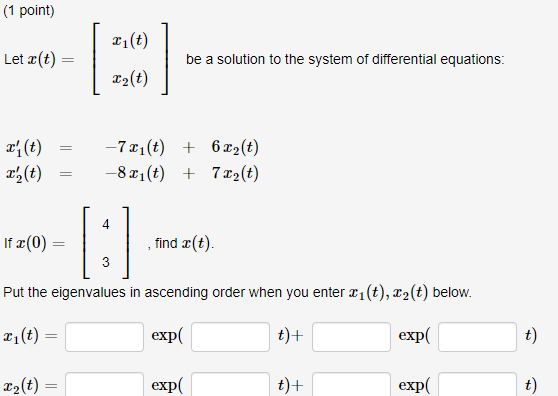(1 point) 21(t) Let z(t) be a solution to the system of differential equations: 22(t) = x(t) xy(t) -7x1(t) + 622(t) -8x1(t) + 722(t) If x(0) find z(t) 3 Put the eigenvalues in ascending order when you enter x1(t), xz(t) below. 21(t) = exp( t)+ exp t) 22(t) exp( t) + exp( t)

• ### Differential Equations. Can someone show a more detailed solution? Having a bit of trouble understanding how to get there with the provided solution. 8. Assume that Xi (t) = (t, 1)T and X2(t) = (t2,...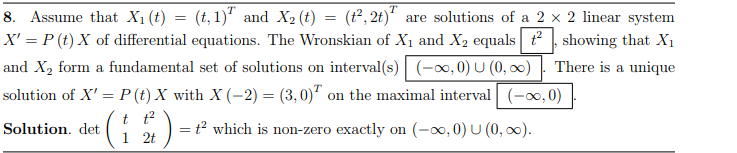Differential Equations. Can someone show a more detailed solution? Having a bit of trouble understanding how to get there with the provided solution. 8. Assume that Xi (t) = (t, 1)T and X2(t) = (t2, 2t)" are solutions of a 2x 2 linear system X, P (t) X of differential equations. The Wronskian of Xi and X2 equals t showing that Xi and X2 form a fundamental set of solutions on interval(s) o,0)U(0,00) There is a unique solution of X...

• ### Consider the linear system of first order differential equations x' = Ax, where x= x(t), t...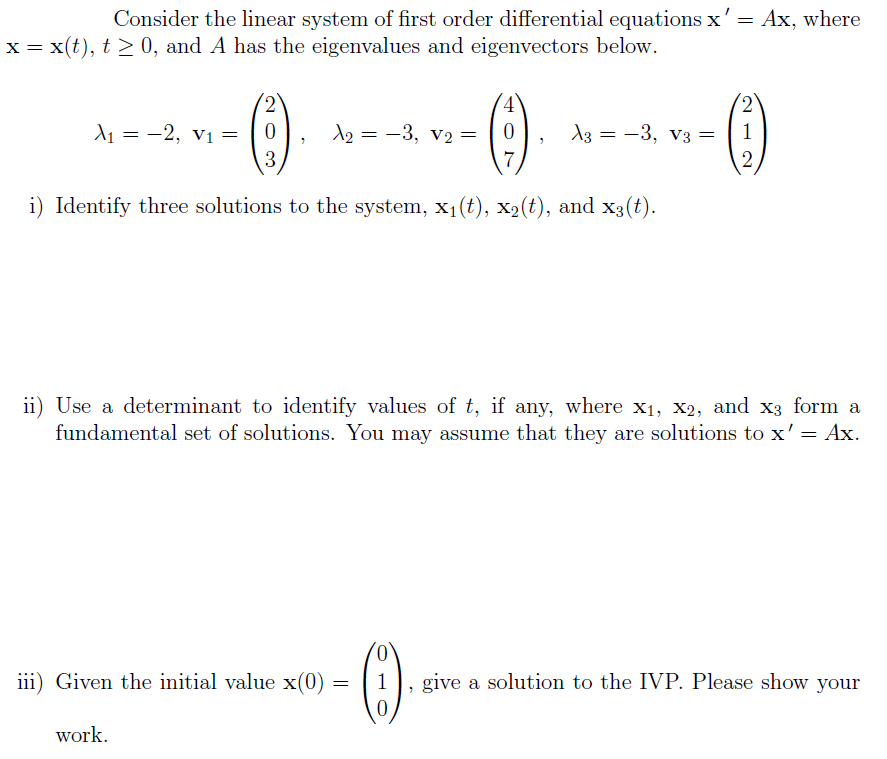Consider the linear system of first order differential equations x' = Ax, where x= x(t), t > 0, and A has the eigenvalues and eigenvectors below. 4 2 11 = -2, V1 = 2 0 3 12 = -3, V2= 13 = -3, V3 = 1 7 2 i) Identify three solutions to the system, xi(t), xz(t), and x3(t). ii) Use a determinant to identify values of t, if any, where X1, X2, and x3 form a fundamental set of...

• ### 6. Solve the system of first order differential equations: x'(t) = X1 + X2 x2(t)x 3x2 6. Solve the system of first order differential equations: x'(t) = X1 + X2 x2(t)x 3x2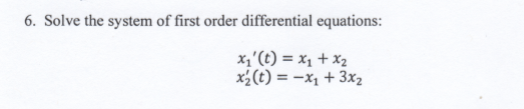6. Solve the system of first order differential equations: x'(t) = X1 + X2 x2(t)x 3x2 6. Solve the system of first order differential equations: x'(t) = X1 + X2 x2(t)x 3x2

• ### Calculate the solution x(t) = (n(t), P2(t),T3(t)) of the system of differential equations X1 = X2...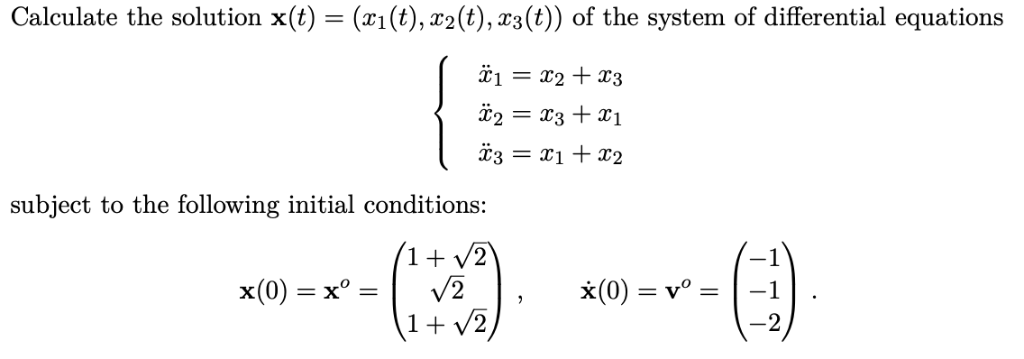Calculate the solution x(t) = (n(t), P2(t),T3(t)) of the system of differential equations X1 = X2 + X3 x3 = x1 + x2 subject to the following initial conditions: Calculate the solution x(t) = (n(t), P2(t),T3(t)) of the system of differential equations X1 = X2 + X3 x3 = x1 + x2 subject to the following initial conditions:

• ### 6. Solve the system of first order differential equations: x'(t) = X1 + X2 x2(t)x 3x26. Solve the system of first order differential equations: x'(t) = X1 + X2 x2(t)x 3x2

• ### х (1 point) Find the solution to the linear system of differential equations . 3.x +...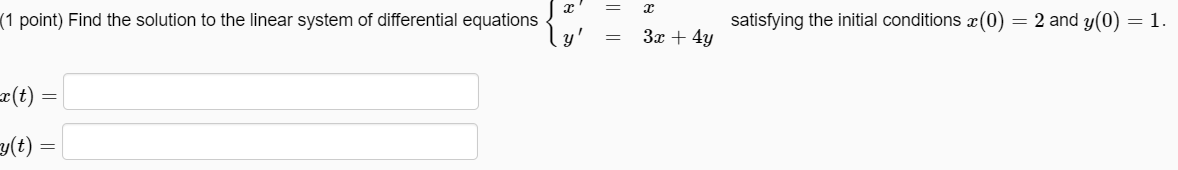х (1 point) Find the solution to the linear system of differential equations . 3.x + 4y satisfying the initial conditions x(0) = 2 and y(0) = 1. = =c(t) = cg(t) =

• ### (1 point) a. Find the most general real-valued solution to the linear system of differential equations...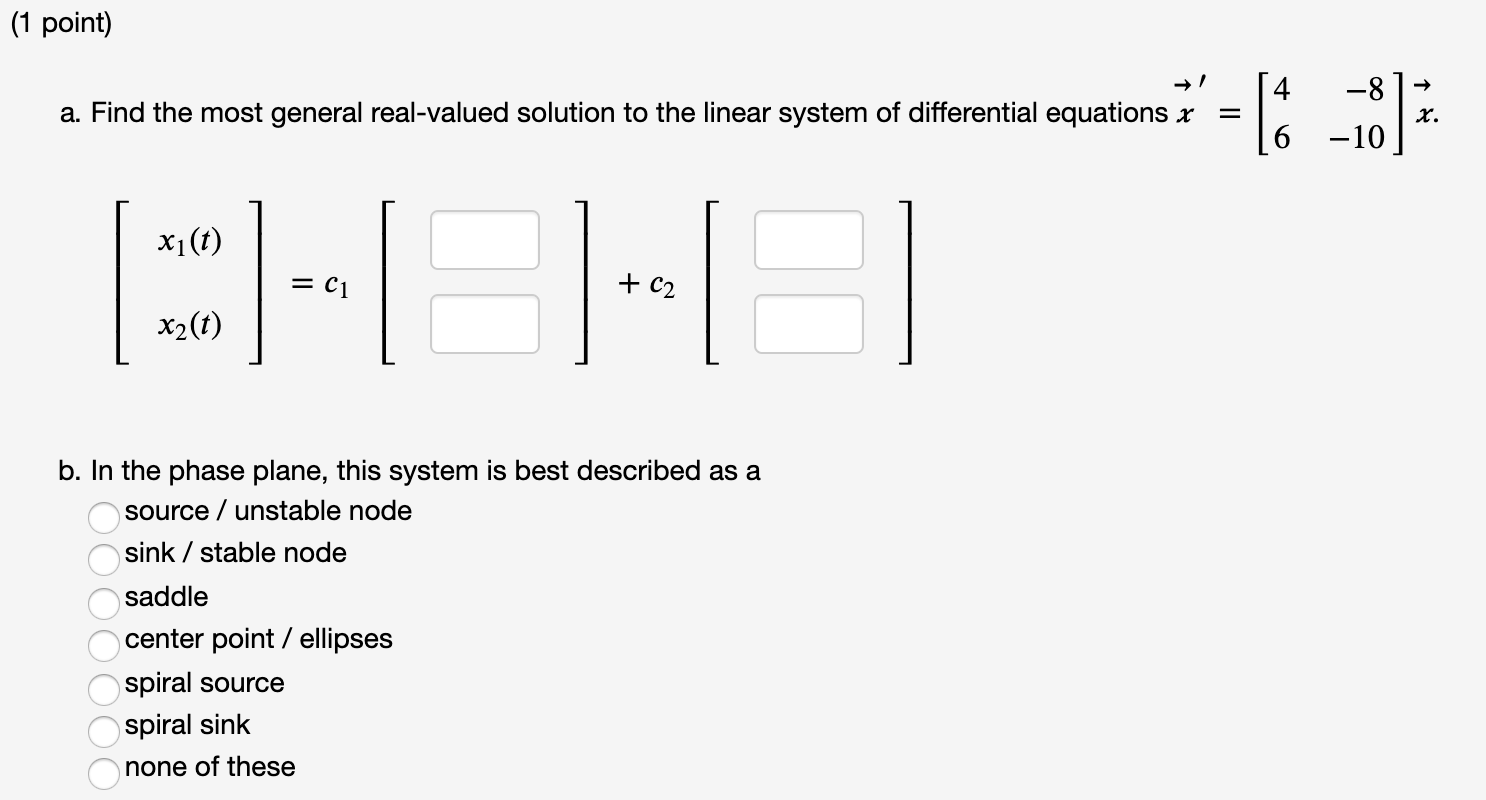(1 point) a. Find the most general real-valued solution to the linear system of differential equations x -8 -10 x. xi(t) = C1 + C2 x2(t) b. In the phase plane, this system is best described as a source / unstable node sink / stable node saddle center point / ellipses spiral source spiral sink none of these ОООООО (1 point) Calculate the eigenvalues of this matrix: [Note-- you'll probably want to use a calculator or computer to estimate the...

• ### Find the most general real-valued solution to the linear system of differential equations (1 point) a. Find the most gen...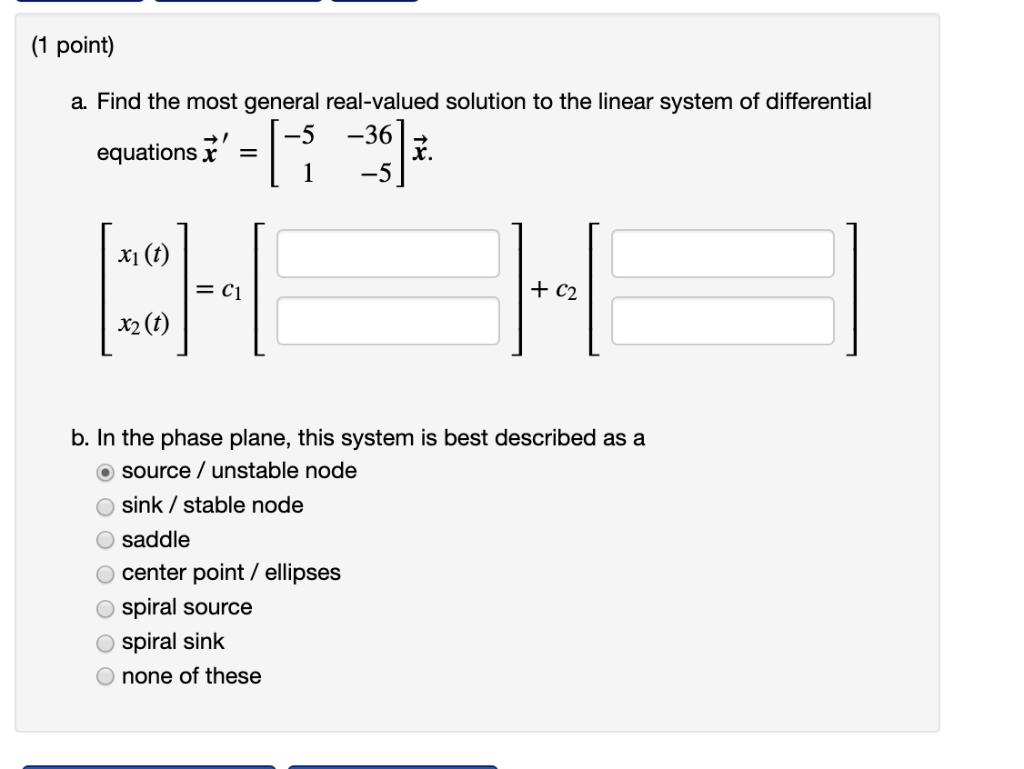Find the most general real-valued solution to the linear system of differential equations (1 point) a. Find the most general real-valued solution to the linear system of differential -5 -36 x. -5 equations x 1 CHH x1 (t) = C1 x2 (t) b. In the phase plane, this system is best described as a O source/ unstable node Osink /stable node Osaddle center point ellipses Ospiral source spiral sink none of these tsi O O O (1 point) a. Find...

Free Homework App